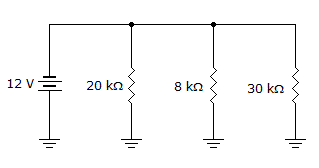# Electronics - Parallel Circuits

### Exercise :: Parallel Circuits - Filling the Blanks

6.

An ammeter is connected in ______ with the branch resistor to measure branch current, and in ______ with the voltage supply to measure total parallel circuit current.

 A. parallel, series B. series, parallel C. series, series D. parallel, parallel

Explanation:

No answer description available for this question. Let us discuss.

7.

Circuit analysis should reveal that the largest resistance in a parallel circuit has the ______.

 A. least current B. most current C. least voltage D. most voltage

Explanation:

No answer description available for this question. Let us discuss.

8.

An open resistor in parallel will cause the total ___ to increase.

 A. voltage B. current C. resistance D. power

Explanation:

No answer description available for this question. Let us discuss.

9.The total power in the given circuit is ___.

 A. 4.8 mW B. 18 mW C. 7.2 mW D. 30 mW

Explanation:

No answer description available for this question. Let us discuss.

10.The total resistance in the given circuit is _____.

 A. greater than 8 kB. 58 kC. 4.8 kD. greater than 30 k